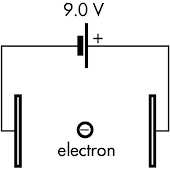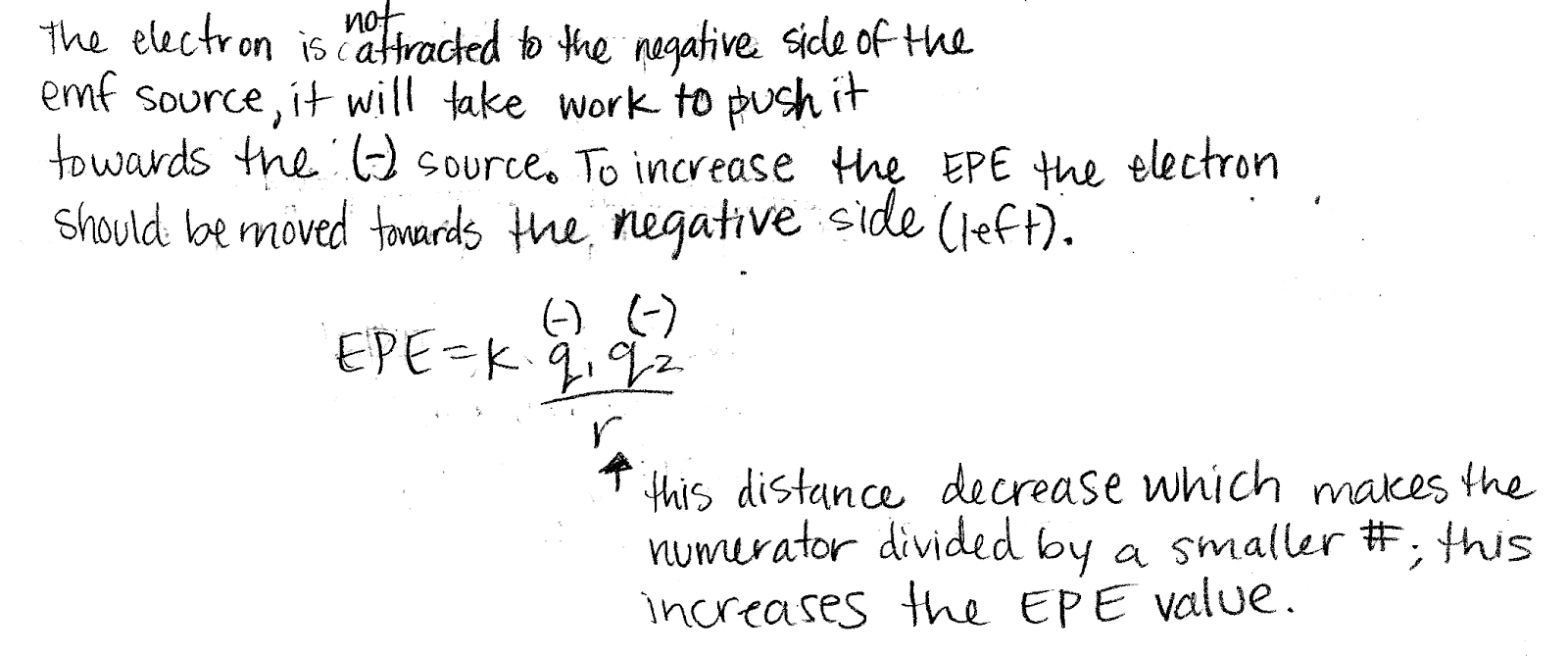## 20170507

### Physics midterm question: electron between capacitor plates

Physics 205B Midterm 2, spring semester 2017
Cuesta College, San Luis Obispo, CAAn electron is placed between the plates of a charged capacitor connected to an ideal 9.0 V emf source. Discuss whether the electron should move to the left or to the right between these plates in order to increase its electric potential energy. Explain your reasoning by using the properties of capacitors, charge, electric potential, and energy.

• p:
Correct. Discusses how the electron would increase its electrical potential energy by moving from right-to-left, by using at least one of the two arguments:
1. the right plate of the capacitor is at a higher potential, and the left plate of the capacitor is at a lower potential, such that a postive charge moving from left-to-right would increase its electric potential energy (ΔU = q⋅ΔV), and thus the negatively charged electron would need to move in the opposite direction (right-to-left) in order to increase its electric potential energy; or
2. the right plate of the capacitor is positively charged, and the left plate of the capacitor is negatively charged, such that the negatively charged electron would require work to be done on it to move right-to-left, bringing it closer to the negative plate (which repels it) while moving farther away from the positive plate (which attracts it).
• r:
As (p), but argument indirectly, weakly, or only by definition supports the statement to be proven, or has minor inconsistencies or loopholes. May not explicitly recognize that the potential difference ΔV applied to the capacitor is constant.
• t:
Nearly correct, but argument has conceptual errors, or is incomplete. Some attempt at applying properties of capacitors, charge, electric potential, and energy.
• v:
Limited relevant discussion of supporting evidence of at least some merit, but in an inconsistent or unclear manner. Some attempt at applying properties of capacitors, charge, electric potential, and energy.
• x:
Implementation/application of ideas, but credit given for effort rather than merit. Approach other than that of applying properties of capacitors, charge, electric potential, and energy.
• y:
Irrelevant discussion/effectively blank.
• z:
Blank.
Sections 30882, 30883
Exam code: midterm02GruT
p: 8 students
r: 9 students
t: 7 students
v: 5 students
x: 0 students
y: 0 students
z: 0 students

A sample "p" response (from student 6969):